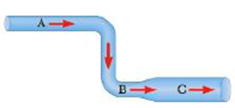Chapter 9, Problem 11CQ

Chapter
Section
Textbook Problem

Water flows along a streamline through the pipe shown in Figure CQ9.11. Point A is higher than points B and C, and the pipe has a constant radius until it expands between B and C. From highest to lowest, (a) rank the flow speeds at points A, B, and C and (b) rank the pressures at points A, B, and C.Figure CQ9.11

(a)

To determine
The flow speeds at points A, B and C.

Explanation

From equation of continuity,

AAvA=ABvB

ABvB=ACvC

The cross sectional areas corresponding to points A and B are the same. Therefore,

AvA=AvBvA=vB

The cross sectional area corresponding to C is greater than that of B

(b)

To determine
The pressure at points A, B and C.

Still sussing out bartleby?

Check out a sample textbook solution.

See a sample solution

The Solution to Your Study Problems

Bartleby provides explanations to thousands of textbook problems written by our experts, many with advanced degrees!

Get Started